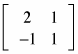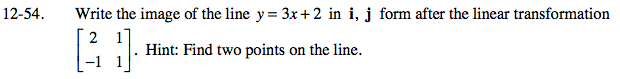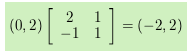### Home > PC > Chapter 12 > Lesson 12.2.2 > Problem12-54

12-54.

Write the image of the line y = 3x + 2 in i, j form after the linear transformation. Hint: Find two points on the line. Homework Help ✎One point on the line is (0, 2). Its image is:Repeat this process for another point on the original line.

Given the points (−2, 2) and (a, b), the vector equation of the line is <−2, 2> + <a, b>t.